# Chapter 14 Practical Geometry Exercise 14.6 Solutions class 6th Ncert

Ncert solutions for Mathematics Class 6th text book Chapter 14 Practical Geometry Exercise 14.6 are given.

Study the textbook lesson Practical Geometry very well.

Observe the example problem and solutions given in the textbook.

Observe the given below solutions and try them in your own method.

You can see Solutions For Maths Class 6

Chapter 1 Knowing our Numbers

Chapter 2 whole Numbers

Some chapters solutions links are given below.

M

# Practical Geometry Solutions Ncert Class 6 Exercise 14.6

Chapter 14 Practical Geometry Exercise 14.6 solutions

Exercise 14.6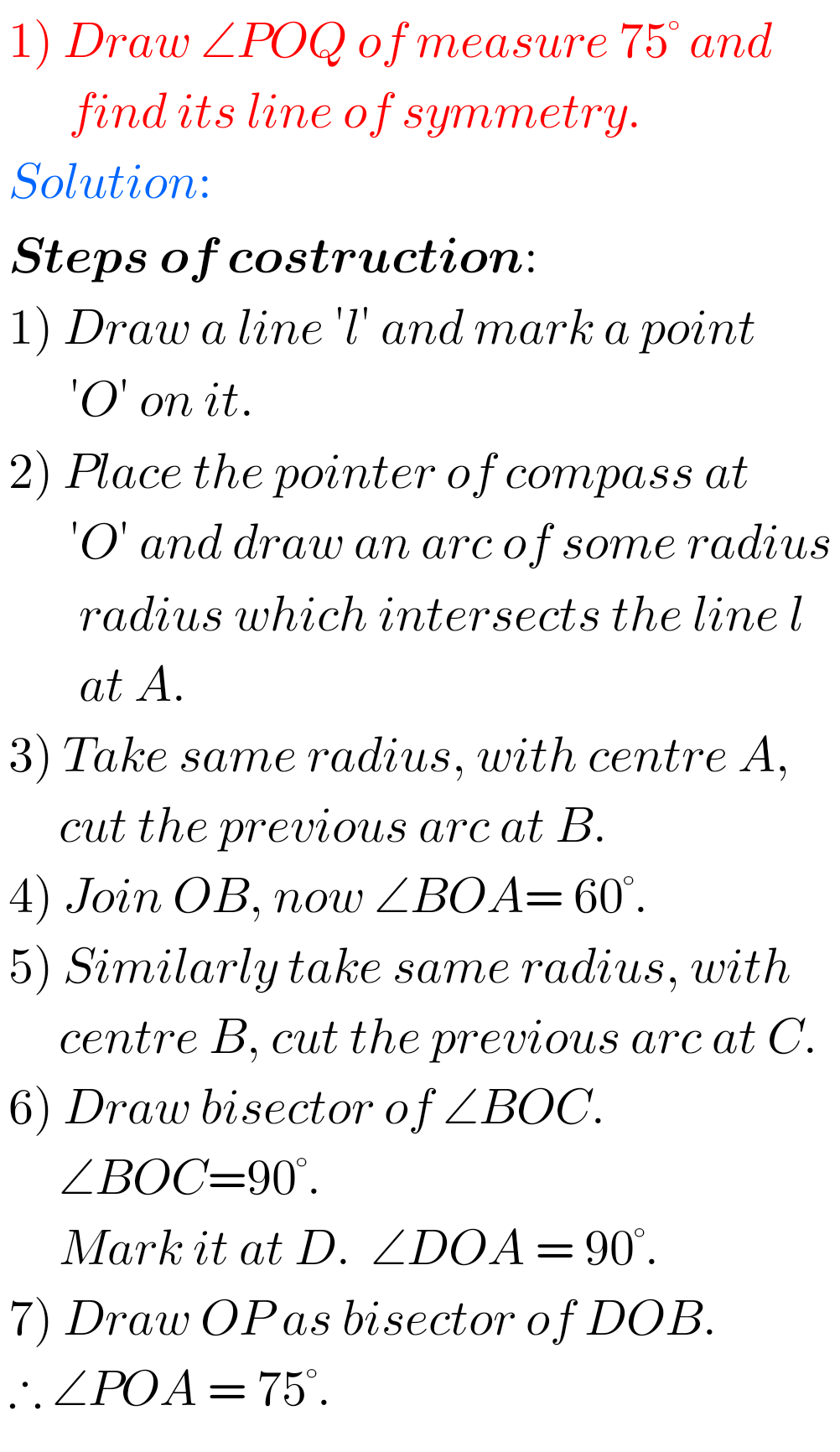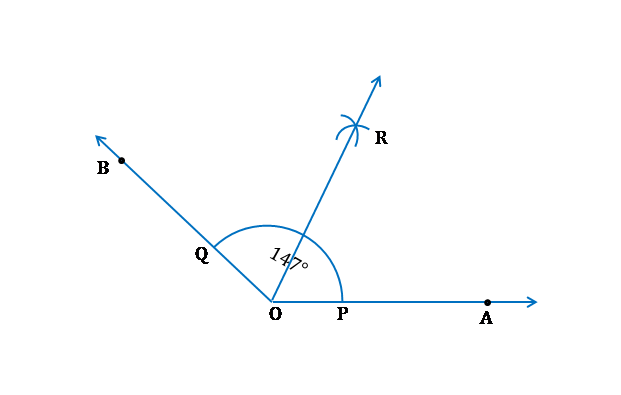## Class 6th Maths Solutions for Practical Geometry Ncert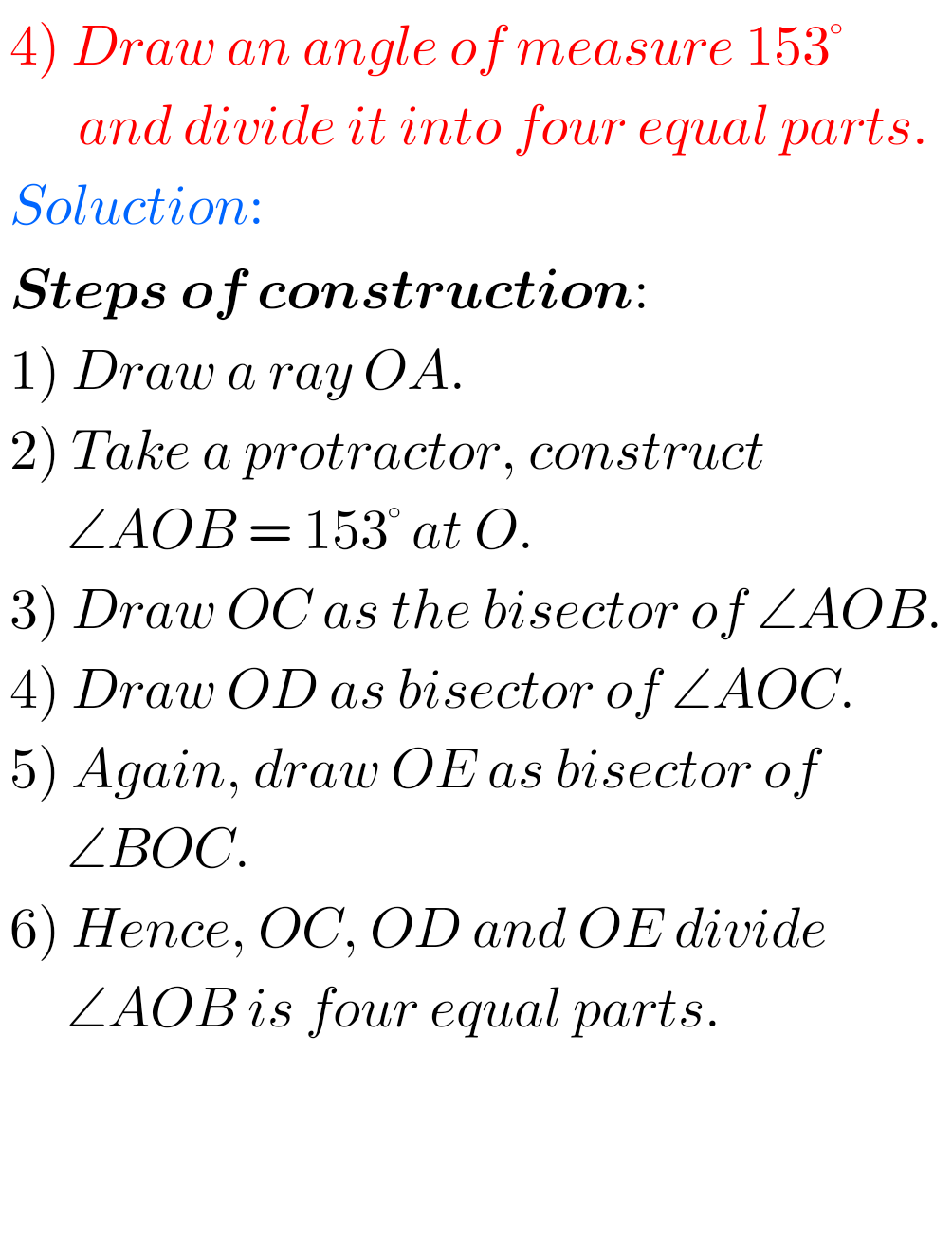## Ncert Solutions for Maths Practical Geometry Class 6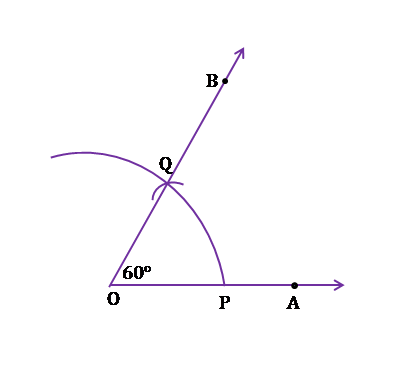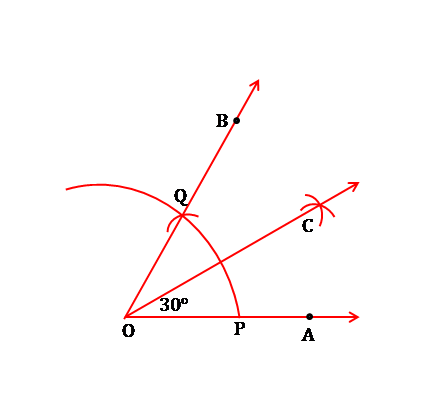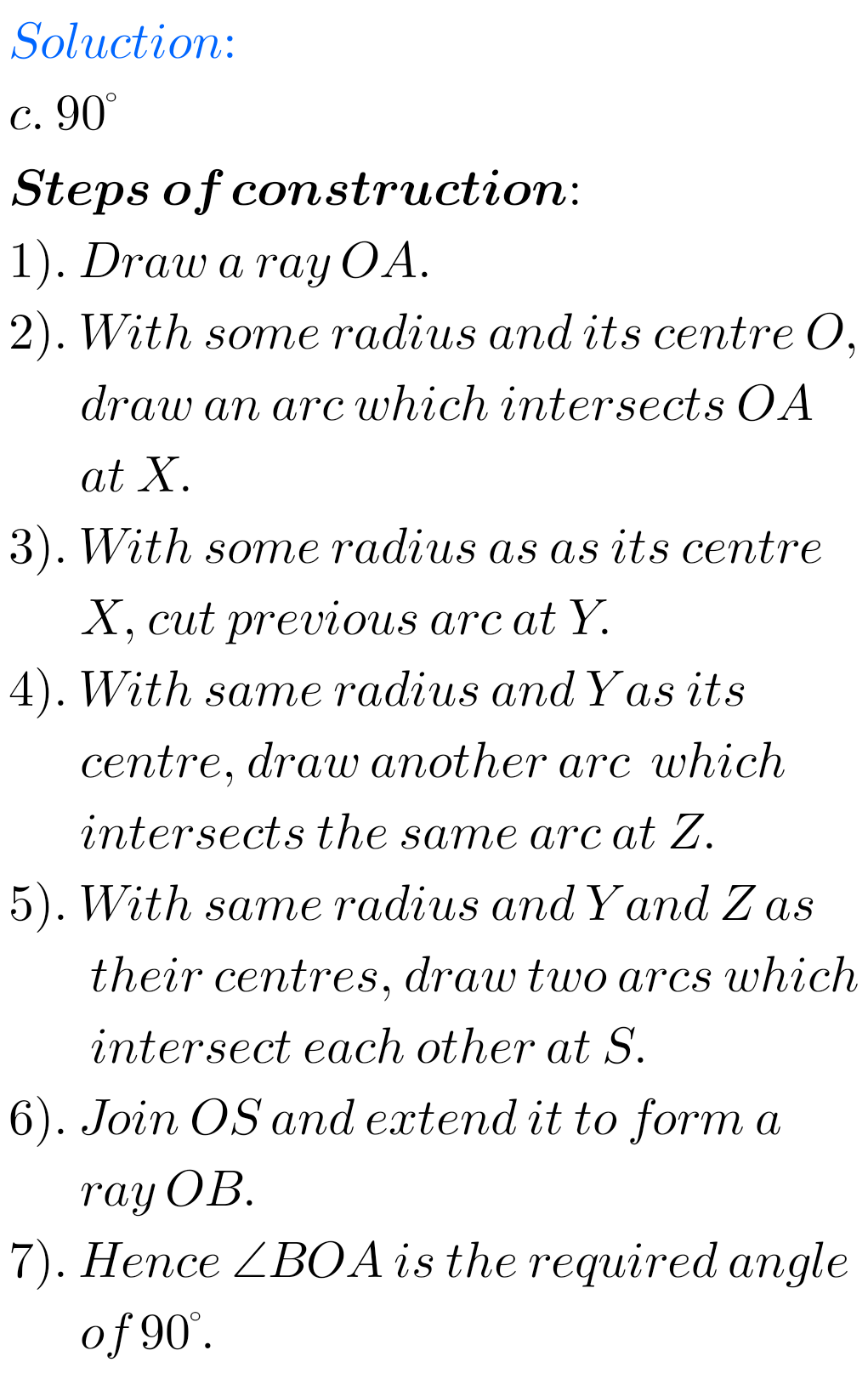F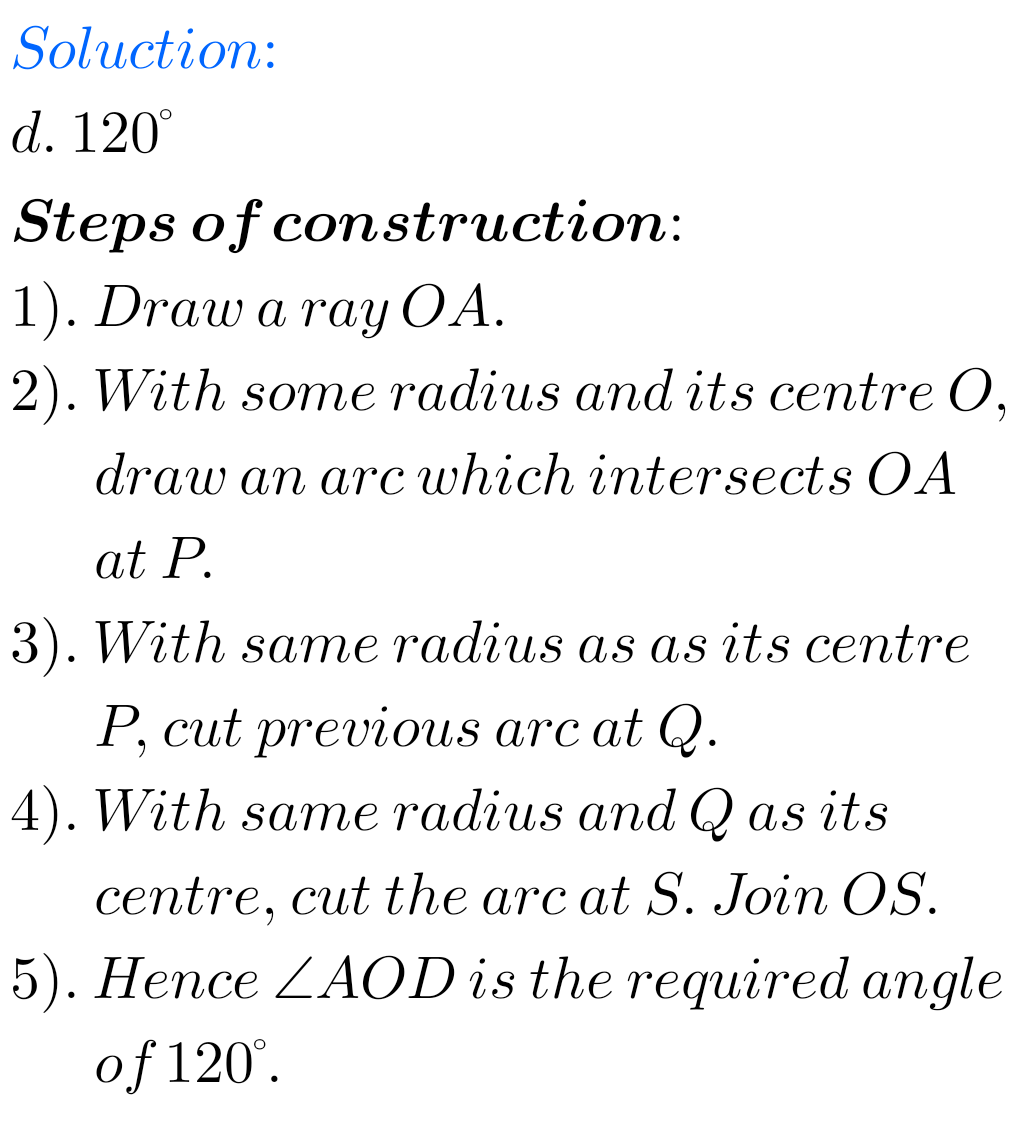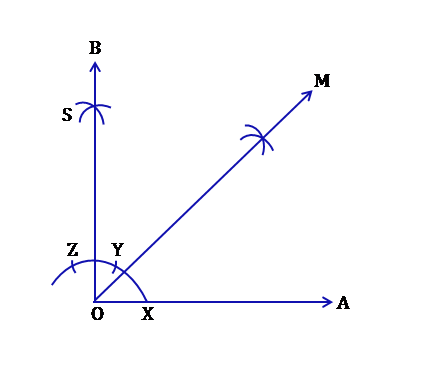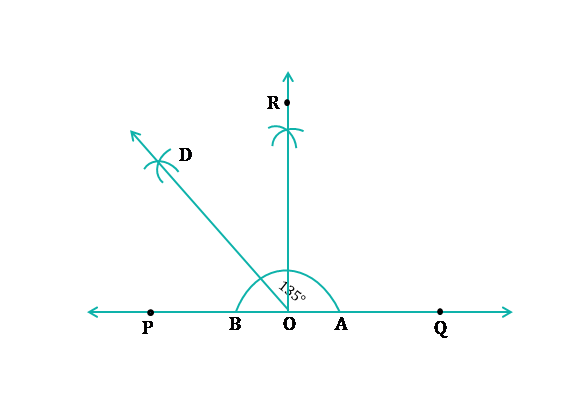### 6th Class Maths Practical Geometry Solutions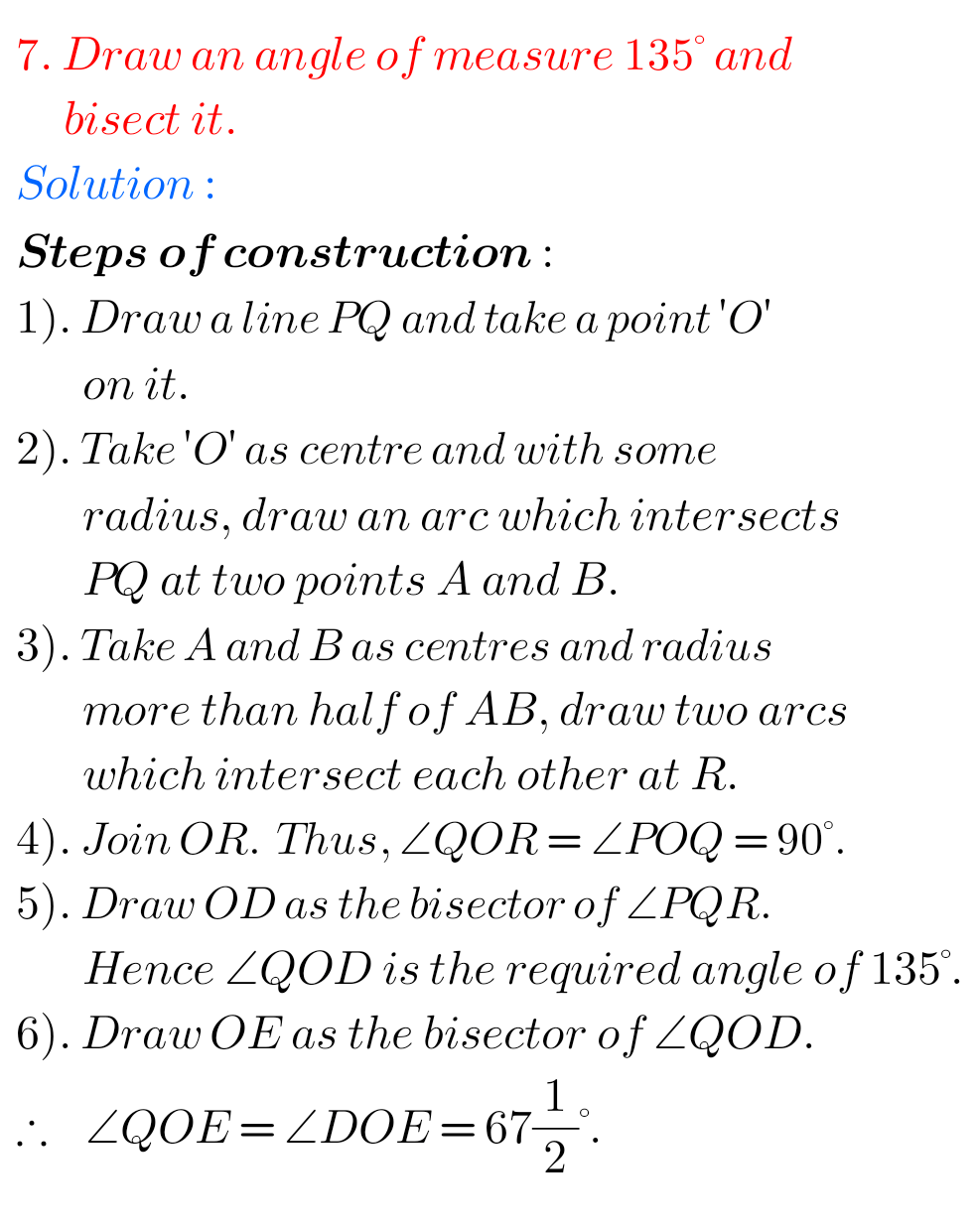FFF

Note : Observe the solutions and try them in your own method.

Chapter 3 Playing with Numbers

Fractions

Chapter 4 Basic Geometrical Ideas

Exercise 4.1

Exercise 4.2

Exercise 4.3

Exercise 4.4

Exercise 4.5

Exercise 4.6

Chapter 6 Integers

Chapter 14 Practical Geometry

Exercise 14.1

Exercise 14.2

Exercise 14.3

Exercise 14.4

Exercise 14.5

Exercise 14.6